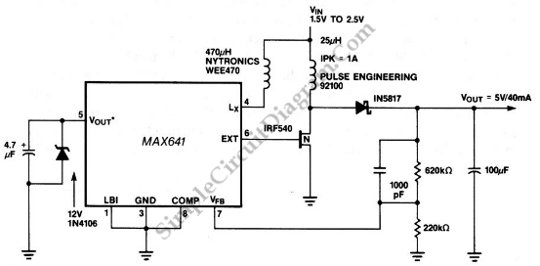# Low-Voltage 1.5V Battery to +5 V DC-DC Converter

Using this circuit a low voltage battery can be increased to 5V. The need of input voltage of this circuit is only 1.5V and the output voltage is 5V at 40mA. Using 2.4V input, this circuit can supply 180mA at 5V. This circuit uses a MAX641 step-up dc/dc converter. The power handling ability and efficiency of MAX641 can be improved by connecting a second inductor to the LX output of a MAX641. Here is the schematic diagram of the circuit: